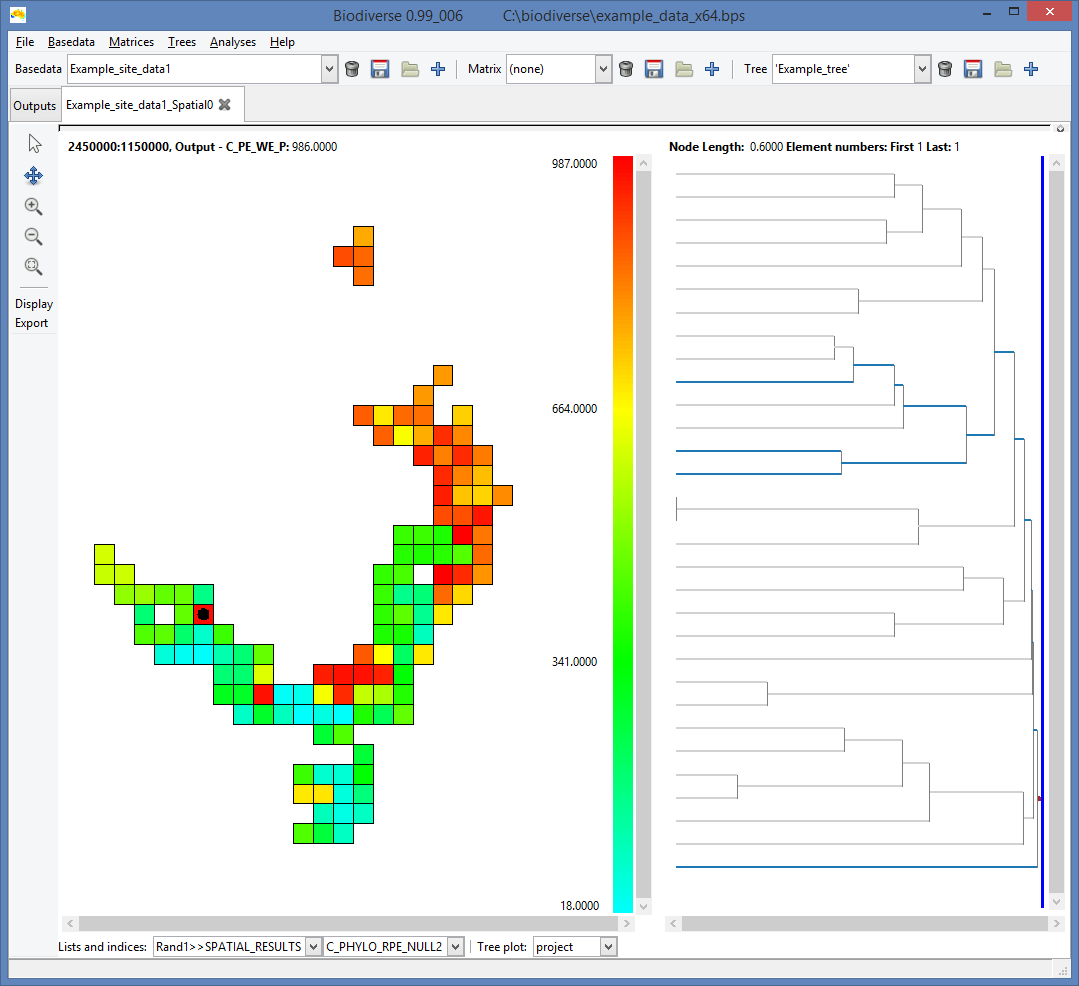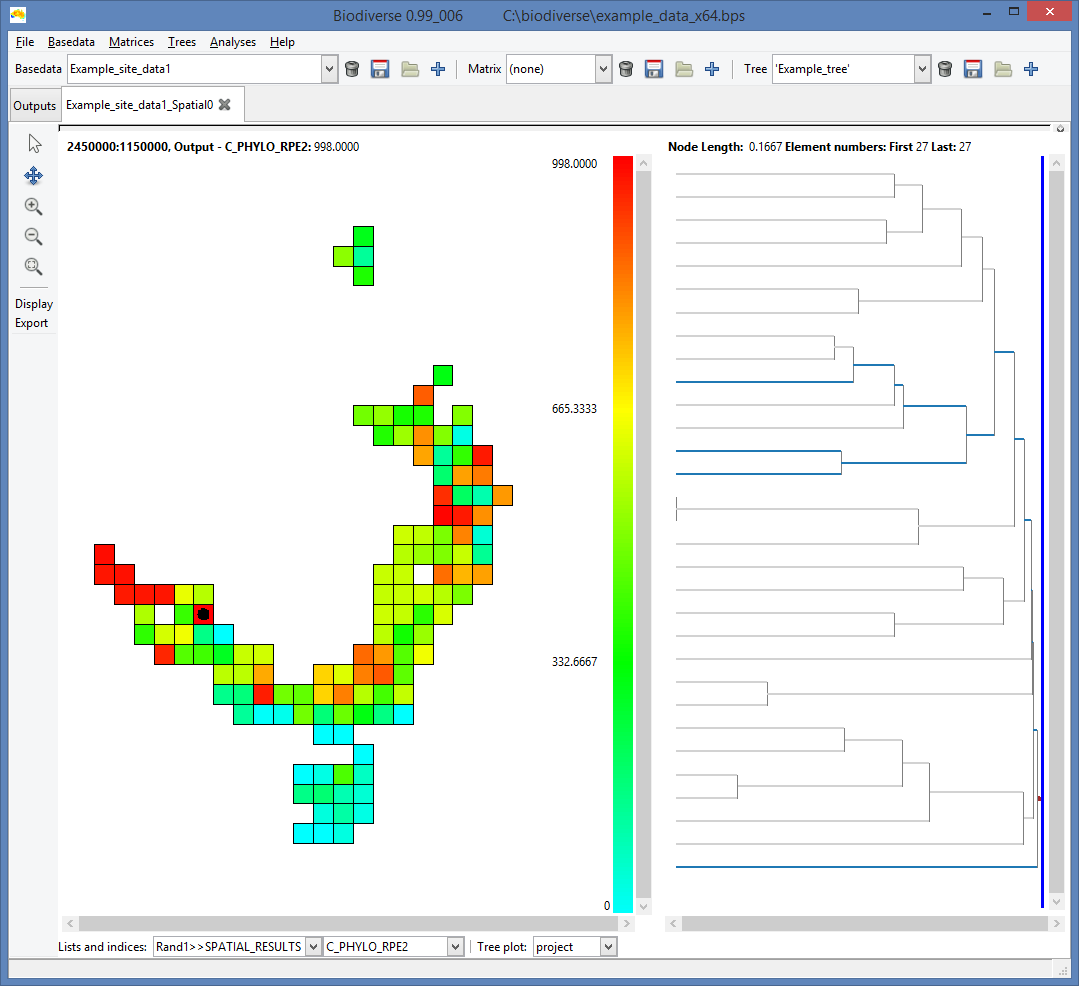## Wednesday, 19 November 2014

### CANAPE - Categorical Analysis of Palaeo and Neo Endemism

[[ Update 2022-10-25:  Biodiverse now calculates CANAPE for you.  See more details in this blog post. ]]

The purpose of this blog is to explain in a bit more detail how the CANAPE method works.  This was prompted by some very useful questions from Stu Marsden.

The paper describing the CANAPE method (Mishler et al. 2014) is at http://dx.doi.org/10.1038/ncomms5473

The CANAPE method is a three step process.  In Step 1 a set of three primary indices as calculated for each region in the data set, in Step 2 a randomisation is run to identify regions with significant endemism.  In Step 3 these regions are classified into palaeo, neo, mixed or non-endemism.

### Step 1.  Calculate the observed endemism

The first step is to calculate a set of three observed endemism scores for each region in the data set:
1. Phylogenetic Endemism (PE) calculated using a user specified tree.  This will be called PE_orig below.
2. PE calculated using an alternate tree.  This will be called PE_alt below.
3. Relative Phylogenetic Endemism (RPE) which is calculated as the ratio of PE_orig to PE_alt.
In this case each region is a single cell, but it could be any collection of cells for which one is interested in running the calculations.

The formula for PE for a region "i" is:whereis the set of branches found in region i,is the local range of branch(the number of cells in region i in which it is found), andis the global range of branch(calculated as the number of cells in which it is found across the whole data set).  Put in words, PE for a region is the sum of the branch lengths found in that region, but where each branch is weighted by the fraction of its geographic range that is found in that region.  It is worth noting that PE is basically is a range-weighted variant of PD (phylogenetic diversity), as the sum of PE scores across all cells will equal the PD for the set of branches found in those cells.

PE_orig and PE_alt are calculated in the same way, the difference is simply in the trees being used.  In Mishler et al. (2014) the alternate tree is one with the same topology as the original tree but where the non-zero branches are modified to be of equal length.  It should be noted that the per-branch range weighting is the same as for PE_orig, so each equalised branch receives the same range weighting as its counterpart in the original tree.

RPE for a region is simply the ratio of PE_orig and PE_alt for that region, so will be >1 when the original tree has longer range weighted branches (PE_orig is longer than on the alternate tree), and <1 when PE_alt has longer range weighted branches (PE_orig is shorter than on the alternate tree). This translates to determining if a region has a collection of longer or shorter range weighted branches.The following plots illustrate the calculation of PE_orig and PE_alt for the example data set that is distributed with Biodiverse.PE_orig (scaled to be proportional to the total tree length).  Branches highlighted in blue are those found in the cell marked with a circle.  (The sum of these branch lengths is the PD of the cell.)  Grey branches are not found in the highlighted cell.  See this blog post for more details about the tree plots)Same as above, but with the tree branches scale to be proportional to their ranges.  PE for the highlighted cell is the sum of these range weighted branches.  It is clear from this plot that the PE in the highlighted cell is largely due to one narrow ranged branch as the other branches in that cell have been considerably downweighted.  (Note also that the branch weighting and thus the weighted lengths will change if collections of cells are used in the analysis, e.g. to calculate the PE of a region instead of a single cell).The map on the left is PE_alt, with the alternate tree on the right (where branches are scaled to be of equal length).  The map colours are not directly comparable between plots due to differing numerical ranges (something to fix in a future version of Biodiverse), but the differing highs and lows are readily differentiated.PE_alt, but now plotting the range weighted version of the equal branch length tree.  Note the similarity with the range weighted tree above for PE_orig, but that it has more detail in the internal branches.

### Step 2.  Use a randomisation to identify regions with significant endemism

The randomisations are needed because we don't have a good basis to directly threshold the values of PE_orig, PE_alt and RPE.  The same value of PE can be obtained by different combinations of terminals (species) as it is a combination of branch lengths and their range weighting, e.g. two long but narrow range branches could be the same as 100 long but wide-ranged branches, so using some predetermined threshold is not likely to be useful.  The same applies to RPE, where the same ratio can arise from different inputs.

The randomisation is done at the level of the species rather than the cell.  In each iteration, species (tree tips) are randomly allocated to the landscape, with the constraint that the richness of each cell is held constant and the same as in the original data set, and thus so is each species' range.  For each random realisation we then calculate PE_orig, PE_alt and RPE for each cell.

We repeat the randomisation 999 times (or more) and keep a track of where the observed PE_orig, PE_alt and RPE for each ell plot against the PE_orig, PE_alt and RPE calculated using the 999 random realisations.  The significance of each cell for each index (PE_orig, PE_alt and RPE) is then the rank relative position of the original values against those of the random realisations, so anything in the top 5% is significantly high for a one tailed test, while anything in the upper 2.5% or lower 2.5% is significant for a two tailed test.

The randomisations are plotted below.  The C_ prefix in the index name means it is the count of times the observed value was greater than the randomised values, so 995 means 995 of the 999 randomisations.  The RPE test is two-tailed so we look for both high and low values (accounting for tied values in the lows, albeit there are none in this example).  These plots don’t have the thresholding applied to them because it is not yet supported in Biodiverse, but it can be done using other tools such as the Biodiverse pipeline or a GIS.Plot of the number of times PE_orig was greater than the same index calculated using the 999 random realisations.Plot of the number of times PE_alt was greater than the same index calculated using the 999 random realisations.Plot of the number of times RPE was greater than the same index calculated using the 999 random realisations.

### Step 3.  Classify the results for each cell

The CANAPE test is then a process of checking the rank relative significance of the PE_orig, PE_alt and RPE values for each cell.  Indents are sub-branches in the decision process.

1)    If either PE_orig or PE_alt are significantly high then we look for palaeo or neo endemism
a)    If RPE is significantly high then we have palaeo-endemism (PE_orig is consistently higher than PE_alt across the random realisations)
b)    Else if RPE is significantly low then we have neo-endemism (PE_orig is consistently lower than PE_alt across the random realisations)
c)    Else we have mixed age endemism in which case
i)    If both PE_orig and PE_alt are highly significant (p<0.01) then we have super endemism (high in both palaeo and neo)
ii)    Else we have mixed (some mixture of palaeo, neo and non endemic)
2)    Else if neither PE_orig or PE_alt are significantly high then we have a non-endemic cell

The bulk of the CANAPE process can be run using the development version of Biodiverse (https://purl.org/biodiverse/wiki/Downloads [Update 2015-04-20 - Version 1 has now been released]).  It is just the final classification which needs external processing, for which there is a pipeline at https://github.com/NunzioKnerr/biodiverse_pipeline but it needs tweaking for the plots to work with other data sets.  The pipeline can actually be used to run the whole thing, it just takes a bit of work to set up at the moment.

Shawn Laffan
19-Nov-2014

For more details about Biodiverse, see http://purl.org/biodiverse

For the full list of changes in the 0.99 series (leading to version 1) see https://purl.org/biodiverse/wiki/ReleaseNotes

To see what else Biodiverse has been used for, see https://purl.org/biodiverse/wiki/PublicationsList
You can also join the Biodiverse-users mailing list at http://groups.google.com/group/Biodiverse-users

Equations are all